inference_wrappers_example

# Inference Wrappers use cases

This is an example of the PySAL segregation framework to perform inference on a single value and comparative inference using simulations under the null hypothesis. Once the segregation classes are fitted, the user can perform inference to shed light for statistical significance in regional analysis. Currently, it is possible to make inference for a single measure or for two values of the same measure.

The summary of the inference wrappers is presented in the following Table:

Inference Type Class/Function Function main Inputs Function Outputs
Single Value SingleValueTest seg_class, iterations_under_null, null_approach, two_tailed p_value, est_sim, statistic
Two Value TwoValueTest seg_class_1, seg_class_2, iterations_under_null, null_approach p_value, est_sim, est_point_diff

Firstly let's import the module/functions for the use case:

%matplotlib inline

import geopandas as gpd
import segregation
import libpysal
import pandas as pd
import numpy as np

from segregation.inference import SingleValueTest, TwoValueTest


Then it's time to load some data to estimate segregation. We use the data of 2000 Census Tract Data for the metropolitan area of Sacramento, CA, USA.

We use a geopandas dataframe available in PySAL examples repository.

s_map = gpd.read_file(libpysal.examples.get_path("sacramentot2.shp"))
s_map.columns

Index(['FIPS', 'MSA', 'TOT_POP', 'POP_16', 'POP_65', 'WHITE_', 'BLACK_',
'ASIAN_', 'HISP_', 'MULTI_RA', 'MALES', 'FEMALES', 'MALE1664',
'FEM1664', 'EMPL16', 'EMP_AWAY', 'EMP_HOME', 'EMP_29', 'EMP_30',
'EMP16_2', 'EMP_MALE', 'EMP_FEM', 'OCC_MAN', 'OCC_OFF1', 'OCC_INFO',
'HH_INC', 'POV_POP', 'POV_TOT', 'HSG_VAL', 'FIPSNO', 'POLYID',
'geometry'],
dtype='object')
gdf = s_map[['geometry', 'HISP_', 'TOT_POP']]


We also can plot the spatial distribution of the composition of the Hispanic population over the tracts of Sacramento:

gdf['composition'] = gdf['HISP_'] / gdf['TOT_POP']

gdf.plot(column = 'composition',
cmap = 'OrRd',
figsize=(20,10),
legend = True)

<matplotlib.axes._subplots.AxesSubplot at 0x20e1272e860>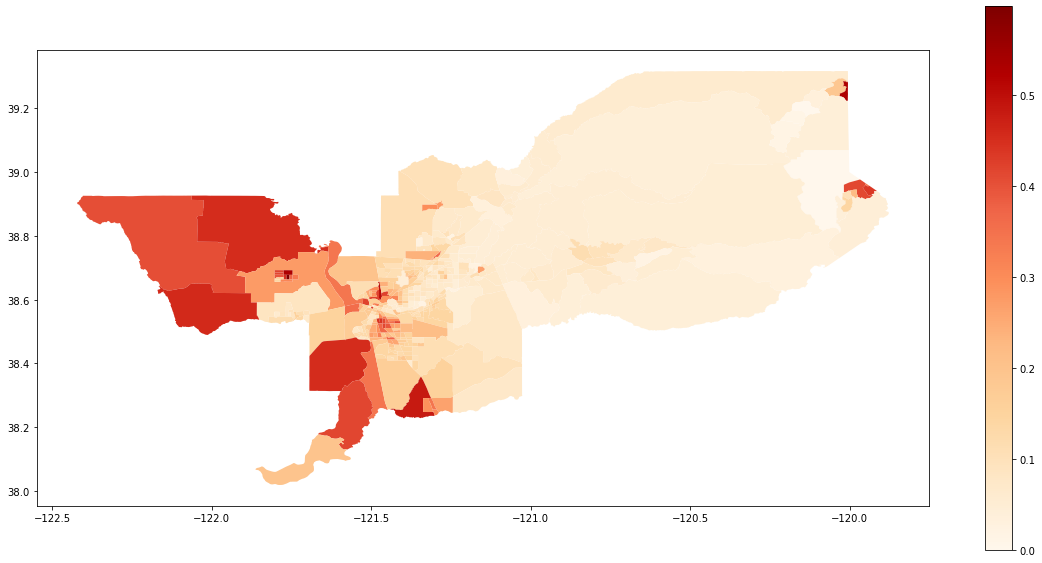## Single Value

### Dissimilarity

The SingleValueTest function expect to receive a pre-fitted segregation class and then it uses the underlying data to iterate over the null hypothesis and comparing the results with point estimation of the index. Thus, we need to firstly estimate some measure. We can fit the classic Dissimilarity index:

from segregation.aspatial import Dissim
D = Dissim(gdf, 'HISP_', 'TOT_POP')
D.statistic

0.32184656076566864

The question that may rise is "Is this value of 0.32 statistically significant under some pre-specified circumstance?". To answer this, it is possible to rely on the Infer_Segregation function to generate several values of the same index (in this case the Dissimilarity Index) under the hypothesis and compare them with the one estimated by the dataset of Sacramento. To generate 1000 values assuming evenness, you can run:

infer_D_eve = SingleValueTest(D, iterations_under_null = 1000, null_approach = "evenness", two_tailed = True)


This class has a quick plotting method to inspect the generated distribution with the estimated value from the sample (vertical red line):

infer_D_eve.plot()

<matplotlib.axes._subplots.AxesSubplot at 0x20e127eb630>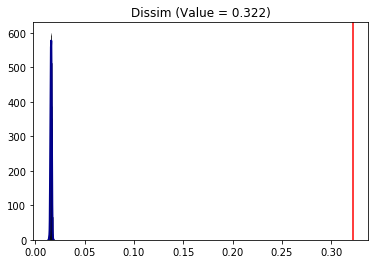It is possible to see that clearly the value of 0.3218 is far-right in the distribution indicating that the hispanic group is, indeed, significantly segregated in terms of the Dissimilarity index under evenness. You can also check the mean value of the distribution using the est_sim attribute which represents all the D draw from the simulations:

infer_D_eve.est_sim.mean()

0.016109671121956267

The two-tailed p-value of the following hypothesis test:

$$H_0: under \ evenness, \ Sacramento \ IS \ NOT \ segregated \ in \ terms \ of \ the \ Dissimilarity \ index \ (D)$$$$H_1: under \ evenness, \ Sacramento \ IS \ segregated \ in \ terms \ of \ the \ Dissimilarity \ index \ (D)$$

can be accessed with the p_value attribute:

infer_D_eve.p_value

0.0

Therefore, we can conclude that Sacramento is statistically segregated at 5% of significance level (p.value < 5%) in terms of D.

You can also test under different approaches for the null hypothesis:

infer_D_sys = SingleValueTest(D, iterations_under_null = 5000, null_approach = "systematic", two_tailed = True)


infer_D_sys.plot()

<matplotlib.axes._subplots.AxesSubplot at 0x20e128235c0>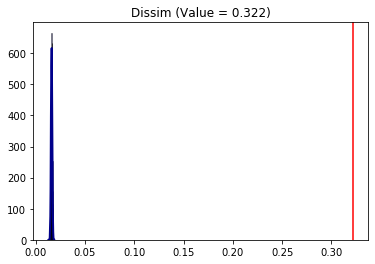The conclusions are analogous as the evenness approach.

### Relative Concentration

The Infer_Segregation wrapper can handle any class of the PySAL segregation module. It is possible to use it in the Relative Concentration (RCO) segregation index:

from segregation.spatial import RelativeConcentration
RCO = RelativeConcentration(gdf, 'HISP_', 'TOT_POP')


Since RCO is an spatial index (i.e. depends on the spatial context), it makes sense to use the permutation null approach. This approach relies on randomly allocating the sample values over the spatial units and recalculating the chosen index to all iterations.

infer_RCO_per = SingleValueTest(RCO, iterations_under_null = 1000, null_approach = "permutation", two_tailed = True)


infer_RCO_per.plot()

<matplotlib.axes._subplots.AxesSubplot at 0x20e15dba9b0>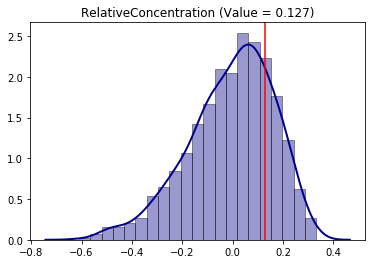infer_RCO_per.p_value

0.452

Analogously, the conclusion for the Relative Concentration index is that Sacramento is not significantly (under 5% of significance, because p-value > 5%) concentrated for the hispanic people.

Additionaly, it is possible to combine the null approaches establishing, for example, a permutation along with evenness of the frequency of the Sacramento hispanic group. With this, the conclusion of the Relative Concentration changes.

infer_RCO_eve_per = SingleValueTest(RCO, iterations_under_null = 1000, null_approach = "even_permutation", two_tailed = True)
infer_RCO_eve_per.plot()


<matplotlib.axes._subplots.AxesSubplot at 0x20e15d8db38>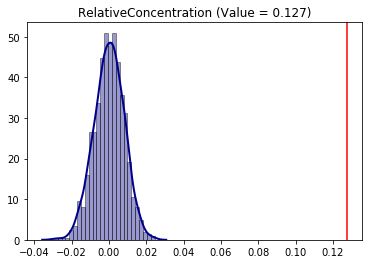### Relative Centralization

Using the same permutation approach for the Relative Centralization (RCE) segregation index:

from segregation.spatial import RelativeCentralization
RCE = RelativeCentralization(gdf, 'HISP_', 'TOT_POP')
infer_RCE_per = SingleValueTest(RCE, iterations_under_null = 1000, null_approach = "permutation", two_tailed = True)

Processed 1000 iterations out of 1000.

infer_RCE_per.plot()The conclusion is that the hispanic group is negatively significantly (as the point estimation is in the left side of the distribution) in terms of centralization. This behavior can be, somehow, inspected in the map as the composition tends to be more concentraded outside of the center of the overall region.

## Comparative Inference

To compare two different values, the user can rely on the TwoValueTest function. Similar to the previous function, the user needs to pass two segregation SM classes to be compared, establish the number of iterations under null hypothesis with iterations_under_null, specify which type of null hypothesis the inference will iterate with null_approach argument and, also, can pass additional parameters for each segregation estimation.

Obs.: in this case, each measure has to be the same class as it would not make much sense to compare, for example, a Gini index with a Delta index

This example uses all census data that the user must provide your own copy of the external database. A step-by-step procedure for downloading the data can be found here: https://github.com/spatialucr/geosnap/blob/master/examples/01_getting_started.ipynb. After the user download the zip files, you must provide the path to these files.

import os
#os.chdir('path_to_zipfiles')

import geosnap

sample = "LTDB_Std_All_Sample.zip"
full = "LTDB_Std_All_fullcount.zip"

read_ltdb(sample = sample, fullcount = full)

df_pre = geosnap.data.db.ltdb

C:\Users\renan\AppData\Local\Continuum\anaconda3\lib\site-packages\pysal\__init__.py:65: VisibleDeprecationWarning: PySAL's API will be changed on 2018-12-31. The last release made with this API is version 1.14.4. A preview of the next API version is provided in the pysal 2.0 prelease candidate. The API changes and a guide on how to change imports is provided at https://pysal.org/about
), VisibleDeprecationWarning)

df_pre.head()

n_asian_under_15 n_black_under_15 n_hispanic_under_15 n_native_under_15 n_white_under_15 n_persons_under_18 n_asian_over_60 n_black_over_60 n_hispanic_over_60 n_native_over_60 ... n_white_persons year n_total_housing_units_sample p_nonhisp_white_persons p_white_over_60 p_black_over_60 p_hispanic_over_60 p_native_over_60 p_asian_over_60 p_disabled
geoid
01001020500 NaN 1.121662 NaN NaN 1.802740 3.284181 NaN 0.301098 NaN NaN ... 5.794934 1970 2.166366 NaN 6.433142 3.514090 NaN NaN NaN 4.737847
01003010100 NaN 609.000000 NaN NaN 639.000000 1407.000000 NaN 221.000000 NaN NaN ... 2003.999981 1970 1106.000000 NaN 8.299712 6.368876 NaN NaN NaN 5.821326
01003010200 NaN 37.567365 NaN NaN 564.014945 686.748041 NaN 27.861793 NaN NaN ... 1757.910752 1970 619.433984 NaN 13.313281 1.480888 NaN NaN NaN 6.248800
01003010300 NaN 374.853457 NaN NaN 981.543199 1523.971872 NaN 103.848314 NaN NaN ... 2835.404427 1970 1025.805309 NaN 8.023381 2.788906 NaN NaN NaN 7.214156
01003010400 NaN 113.203816 NaN NaN 796.944763 1029.919527 NaN 37.127235 NaN NaN ... 2323.133371 1970 780.370269 NaN 11.072073 1.427952 NaN NaN NaN 11.205555

5 rows × 192 columns

In this example, we are interested to assess the comparative segregation of the non-hispanic black people in the census tracts of the Riverside, CA, county between 2000 and 2010. Therefore, we extract the desired columns and add some auxiliary variables:

df = df_pre[['n_nonhisp_black_persons', 'n_total_pop', 'year']]

df['geoid'] = df.index
df['state'] = df['geoid'].str[0:2]
df['county'] = df['geoid'].str[2:5]

n_nonhisp_black_persons n_total_pop year geoid state county
geoid
01001020500 NaN 8.568306 1970 01001020500 01 001
01003010100 NaN 3469.999968 1970 01003010100 01 003
01003010200 NaN 1881.424759 1970 01003010200 01 003
01003010300 NaN 3723.622031 1970 01003010300 01 003
01003010400 NaN 2600.033045 1970 01003010400 01 003

Filtering Riverside County and desired years of the analysis:

df_riv = df[(df['state'] == '06') & (df['county'] == '065') & (df['year'].isin(['2000', '2010']))]

n_nonhisp_black_persons n_total_pop year geoid state county
geoid
06065030101 58.832932 851.999976 2000 06065030101 06 065
06065030103 120.151764 1739.999973 2000 06065030103 06 065
06065030104 367.015289 5314.999815 2000 06065030104 06 065
06065030200 348.001105 4682.007896 2000 06065030200 06 065
06065030300 677.998901 4844.992203 2000 06065030300 06 065

Merging it with desired map.

map_url = 'https://raw.githubusercontent.com/renanxcortes/inequality-segregation-supplementary-files/master/Tracts_grouped_by_County/06065.json'
gdf = map_gpd.merge(df_riv,
left_on = 'GEOID10',
right_on = 'geoid')[['geometry', 'n_nonhisp_black_persons', 'n_total_pop', 'year']]

gdf['composition'] = np.where(gdf['n_total_pop'] == 0, 0, gdf['n_nonhisp_black_persons'] / gdf['n_total_pop'])

gdf.head()

geometry n_nonhisp_black_persons n_total_pop year composition
0 POLYGON ((-117.319414 33.902109, -117.322528 3... 233.824879 2537.096784 2000 0.092162
1 POLYGON ((-117.319414 33.902109, -117.322528 3... 568.000000 6556.000000 2010 0.086638
2 POLYGON ((-117.504056 33.800257, -117.502758 3... 283.439545 3510.681010 2000 0.080736
3 POLYGON ((-117.504056 33.800257, -117.502758 3... 754.000000 10921.000000 2010 0.069041
4 POLYGON ((-117.472451 33.762031, -117.475661 3... 273.560455 3388.318990 2000 0.080736
gdf_2000 = gdf[gdf.year == 2000]
gdf_2010 = gdf[gdf.year == 2010]


Map of 2000:

gdf_2000.plot(column = 'composition',
cmap = 'OrRd',
figsize = (30,5),
legend = True)

<matplotlib.axes._subplots.AxesSubplot at 0x2b4c0812358>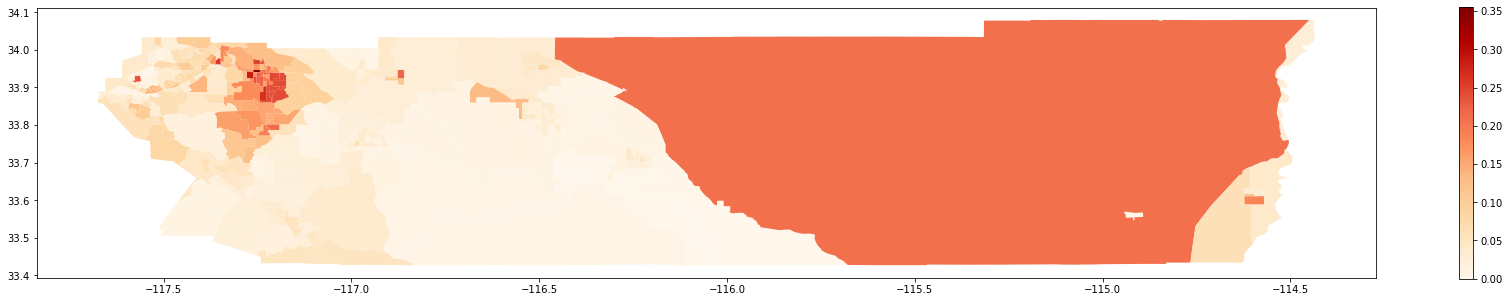Map of 2010:

gdf_2010.plot(column = 'composition',
cmap = 'OrRd',
figsize = (30,5),
legend = True)

<matplotlib.axes._subplots.AxesSubplot at 0x2b48c35f550>A question that may rise is "Was it more or less segregated than 2000?". To answer this, we rely on simulations to test the following hypothesis:

$$H_0: Segregation\ Measure_{2000} - Segregation\ Measure_{2010} = 0$$

### Comparative Dissimilarity

D_2000 = Dissim(gdf_2000, 'n_nonhisp_black_persons', 'n_total_pop')
D_2010 = Dissim(gdf_2010, 'n_nonhisp_black_persons', 'n_total_pop')
D_2000.statistic - D_2010.statistic

0.023696202305264924

We can see that Riverside was more segregated in 2000 than in 2010. But, was this point difference statistically significant? We use the random_label approach which consists in random labelling the data between the two periods and recalculating the Dissimilarity statistic (D) in each iteration and comparing it to the original value.

compare_D_fit = TwoValueTest(D_2000, D_2010, iterations_under_null = 1000, null_approach = "random_label")

Processed 1000 iterations out of 1000.


The TwoValueTest class also has a plotting method:

compare_D_fit.plot()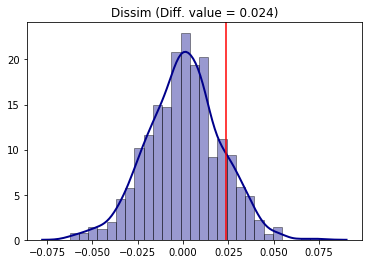To access the two-tailed p-value of the test:

compare_D_fit.p_value

0.26

The conclusion is that, for the Dissimilarity index and 5% of significance, segregation in Riverside was not different between 2000 and 2010 (since p-value > 5%).

### Comparative Gini

Analogously, the same steps can be made for the Gini segregation index.

from segregation.aspatial import GiniSeg
G_2000 = GiniSeg(gdf_2000, 'n_nonhisp_black_persons', 'n_total_pop')
G_2010 = GiniSeg(gdf_2010, 'n_nonhisp_black_persons', 'n_total_pop')
compare_G_fit = TwoValueTest(G_2000, G_2010, iterations_under_null = 1000, null_approach = "random_label")
compare_G_fit.plot()

Processed 1000 iterations out of 1000.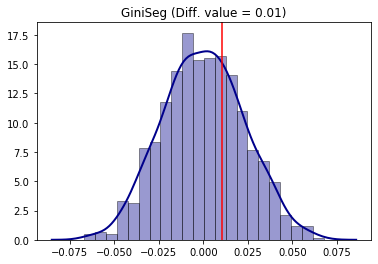The absence of significance is also present as the point estimation of the difference (vertical red line) is located in the middle of the distribution of the null hypothesis simulated.

### Comparative Spatial Dissimilarity

As an example of a spatial index, comparative inference can be performed for the Spatial Dissimilarity Index (SD). For this, we use the counterfactual_composition approach as an example.

In this framework, the population of the group of interest in each unit is randomized with a constraint that depends on both cumulative density functions (cdf) of the group of interest composition to the group of interest frequency of each unit. In each unit of each iteration, there is a probability of 50\% of keeping its original value or swapping to its corresponding value according of the other composition distribution cdf that it is been compared against.

from segregation.spatial import SpatialDissim
SD_2000 = SpatialDissim(gdf_2000, 'n_nonhisp_black_persons', 'n_total_pop')
SD_2010 = SpatialDissim(gdf_2010, 'n_nonhisp_black_persons', 'n_total_pop')
compare_SD_fit = TwoValueTest(SD_2000, SD_2010, iterations_under_null = 500, null_approach = "counterfactual_composition")
compare_SD_fit.plot()

Processed 500 iterations out of 500.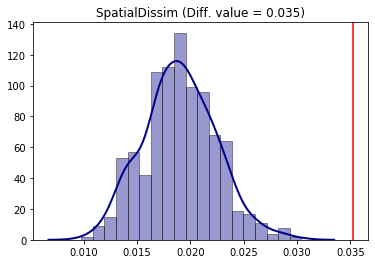The conclusion is that for the Spatial Dissimilarity index under this null approach, the year of 2000 was more segregated than 2010 for the non-hispanic black people in the region under study.